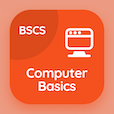Computer Science Online Courses

Digital Logic Design Quizzes

Digital Logic Design Practice Test 8

The e-Book Adder and Subtractors Multiple Choice Questions and Answers (MCQs), adder and subtractors quiz answers PDF chapter 8-8 to study online courses, digital logic design tests. Study DLD Lab Equipment and Experiments MCQ trivia questions, adder and subtractors Multiple Choice Questions (MCQ Quiz) for online college degrees. The e-Book "Adder and Subtractors MCQs" App Download: adder and subtractors, product of sums simplification, boolean functions in dld, shift registers, subtraction with complement test prep for computer software engineer online degree.

The MCQ "One operation that is not given by magnitude comparator is" PDF, Adder and Subtractors App Android & iOS (Free) with less, equal, greater, and addition choices for computer software engineer. Practice dld lab equipment and experiments questions and answers, Google eBook to download free sample to learn online certificate courses.

## Logic Design: Adder & Subtractors MCQ Quiz

MCQ: One operation that is not given by magnitude comparator is

A) equal
B) less
C) greater

MCQ: The output of AND gates in Sum of Product (SOP) is connected to

A) NOT gates
B) OR gates
C) AND gates
D) XOR gates

MCQ: The expression for Absorption law is given by

A) A + AB = A
B) A + AB = B
C) AB + AA' = A
D) A + B = B + A

MCQ: Enable input of the shift register is known as

B) store
C) reset
D) strobe

MCQ: Using 10's complement subtracting 3250 from 72532 is equal to

A) 69282
B) 69272
C) 69252
D) 69232

### Class Quizzes: Digital Logic Design Prep Tests

Download Digital Logic Design Quiz App, Computer Networks MCQs App and Computer Basics MCQ App for Android & iOS devices. These Apps include complete analytics of real time attempts with interactive assessments. Download Play Store & App Store Apps & Enjoy 100% functionality with subscriptions!